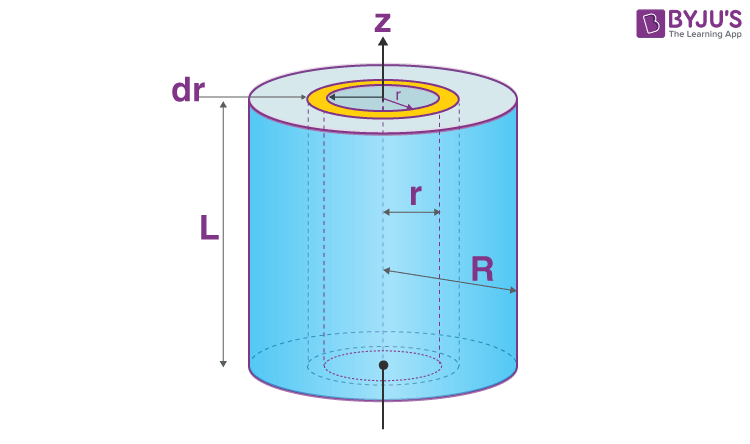Checkout JEE MAINS 2022 Question Paper Analysis : Checkout JEE MAINS 2022 Question Paper Analysis :

# Moment Of Inertia Of A Solid Cylinder

Moment of inertia of a solid cylinder about its centre is given by the formula;

 $$\begin{array}{l}I = \frac{1}{2}MR^{2}\end{array}$$

Here, M = total mass and R = radius of the cylinder.

## Derivation Of Moment Of Inertia Of Solid Cylinder

We will take a solid cylinder with mass M, radius R and length L. We will calculate its moment of inertia about the central axis.Here we have to consider a few things:

• The solid cylinder has to be cut or split into infinitesimally thin rings.
• Each ring consists of the thickness of dr with length L.
• We have to sum up the moments of infinitesimally these thin cylindrical shells.

We will follow the given steps.

1. We will use the general equation of moment of inertia:

dI = r2 dm

Now we move on to finding the dm. It is normally given as;

dm = ρ dV

In order to obtain dm we have to calculate dv first. It is given as;

dV = dA L

Meanwhile, dA is the area of the big ring (radius: r + dr) minus the smaller ring (radius: r). Hence;

$$\begin{array}{l}dA = \pi (r + dr)^{2} – \pi r^{2}\end{array}$$
$$\begin{array}{l}dA = \pi (r^{2}+ 2rdr + (dr)^{2}) – \pi r^{2}\end{array}$$

Notably, here the (dr)2 = 0.

$$\begin{array}{l}dA = 2\pi r dr\end{array}$$

2. Substitution of dA into dV we get;

$$\begin{array}{l}dV = dAL = 2\pi r dr L \end{array}$$

Now, we substitute dV into dm and we will have;

dm = (2πrdr)Lρ

The dm expression is further substituted into the dI equation and we get;

$$\begin{array}{l}dI = \int r^{2}(2\pi rdr)L\rho\end{array}$$
$$\begin{array}{l}I = 2\pi L\rho \int_{0}^{R}r^{3}dr\end{array}$$
$$\begin{array}{l}I = 2\pi L\rho [r^{4}/4]_{0}^{R}\end{array}$$
$$\begin{array}{l}I = 2\pi L\rho [R^{4}/4]\end{array}$$

3. Alternatively, we have to find the expression for density as well. We use the equation;

$$\begin{array}{l}ρ = \frac{M}{V}\end{array}$$

Now,

$$\begin{array}{l}ρ = \frac{M}{\pi R^{2}L}\end{array}$$

4. The final step involves using integration to find the moment of inertia of the solid cylinder. The integration basically takes the form of a polynomial integral form.

$$\begin{array}{l}I = 2\pi L\frac{M}{\pi R^{2}L} [R^{4}/4]\end{array}$$

I = 2MR2/4

Therefore,

$$\begin{array}{l}I = \frac{1}{2}MR^{2}\end{array}$$

## Parallel Axis Theorem